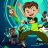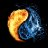# Volatility Based Momentum Indicator for ThinkorSwim#### BenTen

Staff
VIP
The Volatility Based Momentum (VBM) indicator is a variation on the rate-of-change ( ROC ) indicator. Instead of expressing momentum in a percentage gain or loss, VBM normalizes momentum using the historical volatility of the underlying security...The calculation for a volatility based momentum (VBM) indicator is very similar to ROC , but divides by the security’s historical volatility instead. The average true range indicator (ATR) is used to compute historical volatility.

For example, on a daily chart , VBM(22,65) calculates how many MoV price has increased or decreased over the last 22 trading days (approximately one calendar month). The second parameter is the number of periods to use with the ATR indicator to normalize the momentum in terms of volatility.

For more details, there is an article further describing VBM and its applicability versus ROC.### thinkScript Code

Code:
``````declare lower;

input rocPeriod = 22;
input atrPeriod = 65;

def rateOfChange = close - close[rocPeriod];
def normalizationATR = atr(atrPeriod);
def volatilityBasedMomentum = rateOfChange / normalizationATR;

plot VBM = volatilityBasedMomentum;
plot ZeroLine = 0;
ZeroLine.AssignValueColor(GetColor(0));``````

D

#### dhruvchauhan11

##### New member
Can we have buy and sell arrows inside of the momentum indicator? Buy signal above 0 and sell signal below 0

T

#### tomsk

##### Well-known member
VIP
I looked at this request, after adding the snippet that @BenTen posted earlier, the resulting display does look a bit squished. I was thinking that perhaps it might be a better idea to transform this into an upper study so that the arrows can be clearly marked on the chart. However perhaps this might not be a perfect study to use for buy/sell as we see the convergence of the so-called "buy"/"sell" arrows that make the signal not clear and confusing. However if the requester really wishes to see those arrows, here is version 1.1 based on @BenTen original study

Code:
``````# Volatility Based Momentum
# tomsk
# 1.12.2020

# V1.0 - 12.03.2019 - BenTen - Initial release of Volatility Based Momentum study (Lower Study)
# V1.1 - 01.12.2020 - tomsk  - Converted to upper study so that intended signals can be displayed

input rocPeriod = 22;
input atrPeriod = 65;

def rateOfChange = close - close[rocPeriod];
def normalizationATR = atr(atrPeriod);
def volatilityBasedMomentum = rateOfChange / normalizationATR;
def VBM = volatilityBasedMomentum;

plot bull = if VBM crosses above 0 then low * 0.996  else Double.NaN;
plot bear = if VBM crosses below 0 then high * 1.004 else Double.NaN;

bull.SetPaintingStrategy(PaintingStrategy.BOOLEAN_ARROW_UP);
bull.SetDefaultColor(Color.YELLOW);
bull.SetLineWeight(3);

bear.SetPaintingStrategy(PaintingStrategy.BOOLEAN_ARROW_DOWN);
bear.SetDefaultColor(Color.CYAN);
bear.SetLineWeight(3);
# End Volatility Based Momentum``````

••Jorge_Ocampo, Barmarrs and BenTen
D

#### diazlaz

##### Well-known member
2019 Donor
VIP
thanks @tomsk - Quick testing on lower time frames, a value of 15, 39 seems to be a good optimization for lower time frame intraday scalps (e.g. 1 to 3 min TF).#### BenTen

Staff
VIP
@tomsk Thank you for the help.

Y

#### Yoel Pablo

##### New member
Hi Tomsk,

Can you help me convert the lower study to upper study so that the arrows can be clearly marked on the chart with sound alert? I tried to convert the lower study code to upper study but was unable to. Enclosed is the lower code

Code:
``````#----Momentum
Declare lower;

input length = 12;
input price = close;
input showBreakoutSignals = no;

Assert(length > 0, "'length' must be positive: " + length);

plot Momentum = price - price[length];
plot ZeroLine = 0;
plot UpSignal = if Momentum crosses above ZeroLine then ZeroLine else Double.NaN;
plot DownSignal = if Momentum crosses below ZeroLine then ZeroLine else Double.NaN;

UpSignal.SetHiding(!showBreakoutSignals);
DownSignal.SetHiding(!showBreakoutSignals);

Momentum.SetDefaultColor(GetColor(1));
ZeroLine.SetDefaultColor(GetColor(0));
UpSignal.SetDefaultColor(Color.UPTICK);
UpSignal.SetPaintingStrategy(PaintingStrategy.ARROW_UP);
DownSignal.SetDefaultColor(Color.DOWNTICK);
DownSignal.SetPaintingStrategy(PaintingStrategy.ARROW_DOWN);

.... and the other one is the code I tried to convert to upper study.

Code:
``````#---Momentum_Upper

input length = 12;
input price = close;

Assert(length > 0, "'length' must be positive: " + length);

def Momentum = price - price[length];
def ZeroLine = 0;

plot UpSignal = if Momentum crosses above ZeroLine then ZeroLine else Double.NaN;
UpSignal.SetDefaultColor(Color.UPTICK);
UpSignal.SetPaintingStrategy(PaintingStrategy.ARROW_UP);

plot DownSignal = if Momentum crosses below ZeroLine then ZeroLine else Double.NaN;
DownSignal.SetDefaultColor(Color.DOWNTICK);
DownSignal.SetPaintingStrategy(PaintingStrategy.ARROW_DOWN);

I truly appreciate your time and effort. Thank you!

Kindly,
YP

Last edited by a moderator:#### horserider

##### Well-known member
VIP
Check logic here.
plot UpSignal = if Momentum crosses above ZeroLine then ZeroLine else Double.NaN;
plot DownSignal = if Momentum crosses below ZeroLine then ZeroLine else Double.NaN;

and boolean arrows

Y

#### Yoel Pablo

##### New member
Hi Horserider,
Thank you for your reply. It helped me in looking at the right direction for the arrow signal. I have a problem with the graphic though. The candlesticks are flatten and the signal arrows are at the botttom of the graph. Do you have any idea on how to fix this?

L

##### New member
Not sure how to load the code into ToS to get it to work, each time I try it does not load it. Any assistance would be greatly appreciated.#### horserider

##### Well-known member
VIP
@Yoel Pablo Did you fix the what I suggested previously? Hard to know the problem without seeing your code.

Y

Hi Horserider,

Last edited:
K

#### KeinFlitter

##### New member
Hi everyone! I have recently registered, but have been following the community for some time now. Thank you for all the great ideas you share! I have an idea regarding this Momentum indicator: Do you think it's possible to calculate the gamma of the displayed value of this indicator? That way it will show a "rate of change" and show an acceleration of momentum. I'm not sure what formula to use for this though.

Later this can be put into a tab so stocks can be sorted by the "momentum rate of change" which will be your trigger.ATR Volatility Based System Indicator for ThinkorSwim Custom 304 & 20 Period Historical Volatility - Reversals and Trend Exhaustion Indicators 0